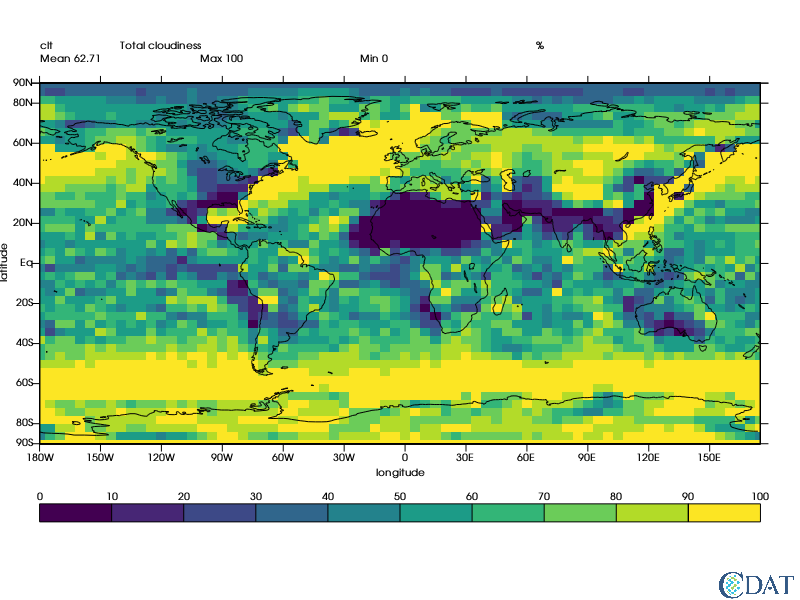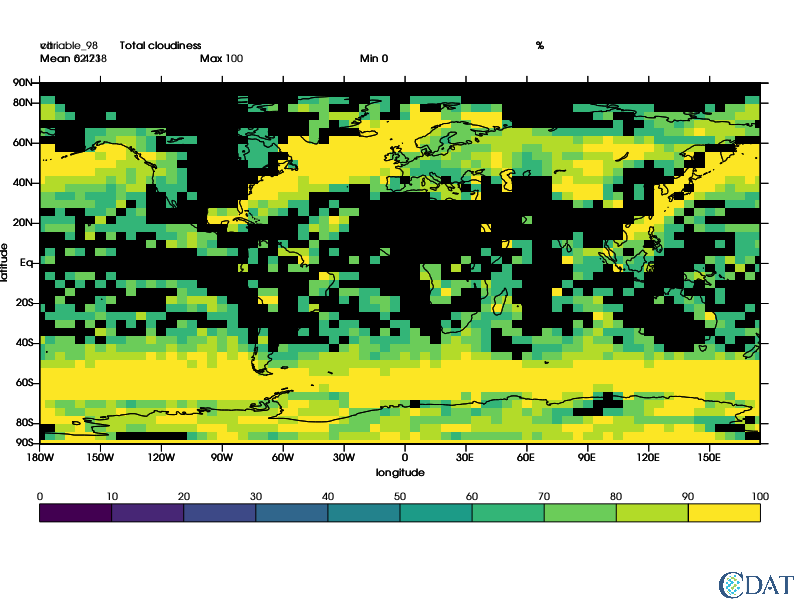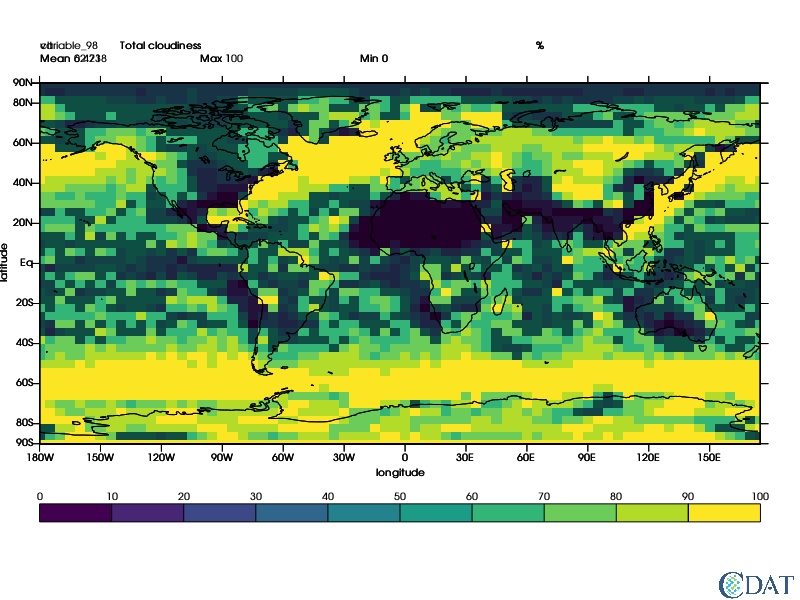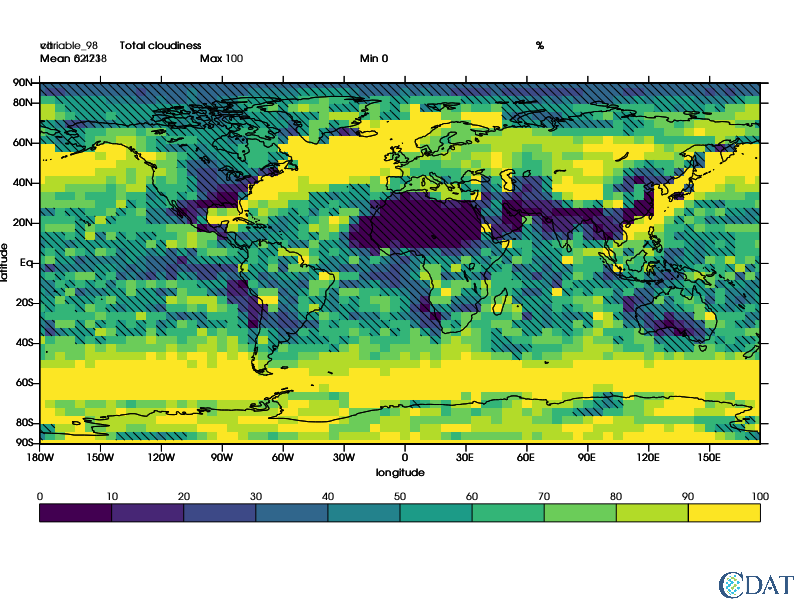# Shading Using Patterns in VCS¶

This notebook shows how to use patterns in vcs.

Pattern can be used with isofill, boxfill, meshfill and fillarea object.

In this notebook we are using primirarly boxfill

The CDAT software was developed by LLNL. This tutorial was written by Charles Doutriaux. This work was performed under the auspices of the U.S. Department of Energy by Lawrence Livermore National Laboratory under Contract DE-AC52-07NA27344.

# Prepare Notebook Elements¶

In :
import requests
r = requests.get("https://uvcdat.llnl.gov/cdat/sample_data/clt.nc",stream=True)
with open("clt.nc","wb") as f:
for chunk in r.iter_content(chunk_size=1024):
if chunk:  # filter local_filename keep-alive new chunks
f.write(chunk)

import cdms2
f = cdms2.open("clt.nc")
clt = f("clt",time=slice(0,1),squeeze=1) # Get first month

/software/anaconda53/envs/cdms2/lib/python3.7/site-packages/numpy/ma/core.py:3174: FutureWarning: Using a non-tuple sequence for multidimensional indexing is deprecated; use arr[tuple(seq)] instead of arr[seq]. In the future this will be interpreted as an array index, arr[np.array(seq)], which will result either in an error or a different result.
dout = self.data[indx]


# Create default Graphic Method¶

In :
import vcs
import cdms2
x=vcs.init(bg=True, geometry=(800,600))
gm = vcs.createboxfill()
gm.boxfill_type = "custom"

In :
# Let's look at the data w/o pattern
x.plot(clt,gm)

Out:Now let's assume we are only interested in areas where clt is greater than 60% let's shade out areas where clt is < 60%

In :
import MV2


In :
# let's create a second boxfill method
gm2 = vcs.createboxfill()
gm2.boxfill_type = "custom"
# and a template for it
tmpl2 = vcs.createtemplate()
tmpl2.legend.priority=0
gm2.levels = [[0.5,1.]]
gm2.fillareacolors = ["black",]

Out:# Method 2: Using Opacity¶

Let's use some opacity to "see" what's bellow

In :
gm2.fillareaopacity = 
x.clear()
x.plot(clt,gm)

Out:# Method 3: Using Patterns¶

Rather than opacity, we can use patterns, that let us see better what's "underneath"

In :
gm2.fillareastyle = "pattern"
gm2.fillareaindices = 
gm2.fillareaopacity = 
x.clear()
x.plot(clt,gm)# we can control the size of patterns